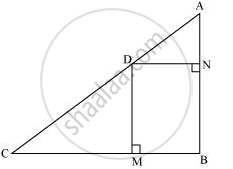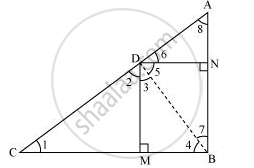Share

# In the given figure, D is a point on hypotenuse AC of ΔABC, DM ⊥ BC and DN ⊥ AB, Prove that: (i) DM2 = DN.MC (ii) DN2 = DM.AN - CBSE Class 10 - Mathematics

ConceptCriteria for Similarity of Triangles

#### Question

In the given figure, D is a point on hypotenuse AC of ΔABC, DM ⊥ BC and DN ⊥ AB, Prove that:

(i) DM2 = DN.MC

(ii) DN2 = DM.AN#### Solution

(i)Let us join DB.We have, DN || CB, DM || AB, and ∠B = 90°

∴ DMBN is a rectangle.

∴ DN = MB and DM = NB

The condition to be proved is the case when D is the foot of the perpendicular drawn from B to AC.

∴ ∠CDB = 90°

⇒ ∠2 + ∠3 = 90° … (1)

In ΔCDM,

∠1 + ∠2 + ∠DMC = 180°

⇒ ∠1 + ∠2 = 90° … (2)

In ΔDMB,

∠3 + ∠DMB + ∠4 = 180°

⇒ ∠3 + ∠4 = 90° … (3)

From equation (1) and (2), we obtain

∠1 = ∠3

From equation (1) and (3), we obtain

∠2 = ∠4

In ΔDCM and ΔBDM,

∠1 = ∠3 (Proved above)

∠2 = ∠4 (Proved above)

∴ ΔDCM ∼ ΔBDM (AA similarity criterion)

=> (BM)/(DM) = (DM)/(MC)

=> (DN)/(DM) = (DM)/(MC)          (BM = DN)

⇒ DM2 = DN × MC

(ii) In right triangle DBN,

∠5 + ∠7 = 90° … (4)

In right triangle DAN,

∠6 + ∠8 = 90° … (5)

D is the foot of the perpendicular drawn from B to AC.

⇒ ∠5 + ∠6 = 90° … (6)

From equation (4) and (6), we obtain

∠6 = ∠7

From equation (5) and (6), we obtain

∠8 = ∠5

In ΔDNA and ΔBND,

∠6 = ∠7 (Proved above)

∠8 = ∠5 (Proved above)

∴ ΔDNA ∼ ΔBND (AA similarity criterion)

=> AN/DN = DN/NB

⇒ DN2 = AN × NB

⇒ DN2 = AN × DM (As NB = DM)

Is there an error in this question or solution?

#### Video TutorialsVIEW ALL 

Solution In the given figure, D is a point on hypotenuse AC of ΔABC, DM ⊥ BC and DN ⊥ AB, Prove that: (i) DM2 = DN.MC (ii) DN2 = DM.AN Concept: Criteria for Similarity of Triangles.
S